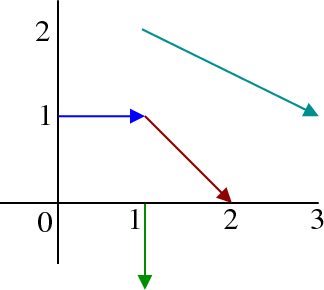# Math Insight

### Image: Sample vectors from a vector fieldA few sample vectors of $\dlvf(x,y)=(y,-x)$ are plotted to illustrate how to represent a vector field.

Image file: vector_field_sample_vectors.png